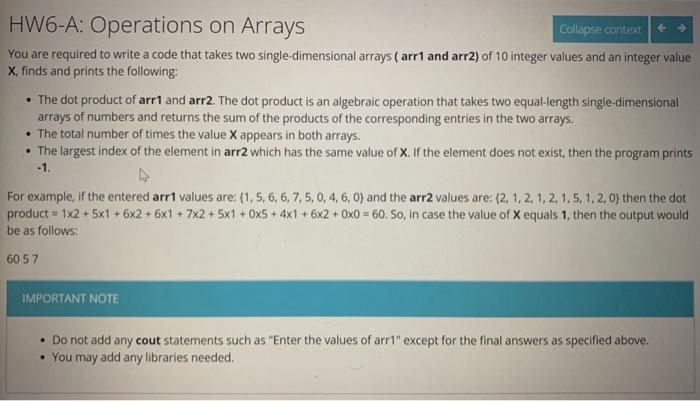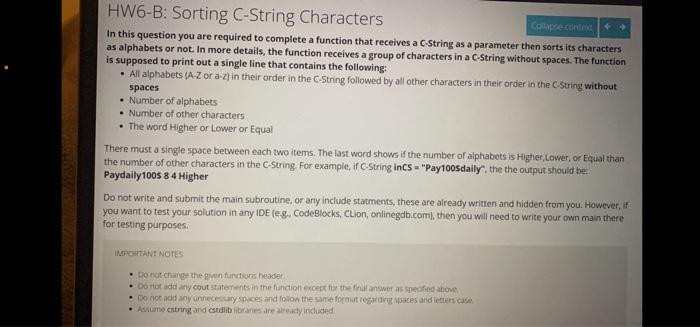Home / Expert Answers / Computer Science / solve-it-in-c-you-are-required-to-write-a-code-that-takes-two-single-dimensional-arrays-oper-pa740

# (Solved): Solve it in C++ You are required to write a code that takes two single-dimensional arrays ( $$\oper ... Solve it in C++You are required to write a code that takes two single-dimensional arrays ( \( \operatorname{arr} 1$$ and arr2) of 10 integer values and an integer value $$X$$, finds and prints the following: - The dot product of arr1 and arr2. The dot product is an algebraic operation that takes two equal-length single-dimensional arrays of numbers and returns the sum of the products of the corresponding entries in the two arrays. - The total number of times the value $$\mathrm{X}$$ appears in both arrays. - The largest index of the element in arr2 which has the same value of $$\mathrm{X}$$. If the element does not exist, then the program prints -1. For example, if the entered arr 1 values are: $$\{1,5,6,6,7,5,0,4,6,0\}$$ and the arr2 values are: $$\{2,1,2,1,2,1,5,1,2,0\}$$ then the dot product $$=1 \times 2+5 \times 1+6 \times 2+6 \times 1+7 \times 2+5 \times 1+0 \times 5+4 \times 1+6 \times 2+0 \times 0=60$$. So, in case the value of $$X$$ equals 1 , then the output would be as follows: 6057 IMPORTANT NOTE - Do not add any cout statements such as "Enter the values of arr1" except for the final answers as specified above. - You may add any libraries needed. HW6-B: Sorting C-String Characters In this question you are required to complete a function that receives a c-String as a parameter then sorts its characters as alphabets or not. In more details, the function receives a group of characters in a C-String without spaces. The function is supposed to print out a single line that contains the following: - All alphabets (A.Z or a.z) in their order in the C-String followed by all other characters in their order in the C.String without spaces - Number of alphabets - Number of other characters - The word Higher or Lower or Equal There must a single space between each two items, The last word shows if the number of alphabets is Higher, Lower, or Equal than the number of other characters in the C-String. For example, if C.String inCS a "Pay100sdaily", the the output should be: Paydaily100s 84 Higher Do not write and submit the main subroutine, or any include statments, these are aiready written and hidden from you. However, if you want to test your solution in any IDE (e.g., CodeBlocks, CLion, onlinegdbicom, then you will need to write your own main there for testing purposes. ismontANR NOIES - Do not change the given functoris header. - Do not add ary cout saternents in the fundion excepe fira the tinalinswer as spected abover - Do not add any unnecesary pices and follow the same format cesarding spaces and ietelest cise - Assuame estring and cstalib libranes are atready inchustid.

We have an Answer from Expert